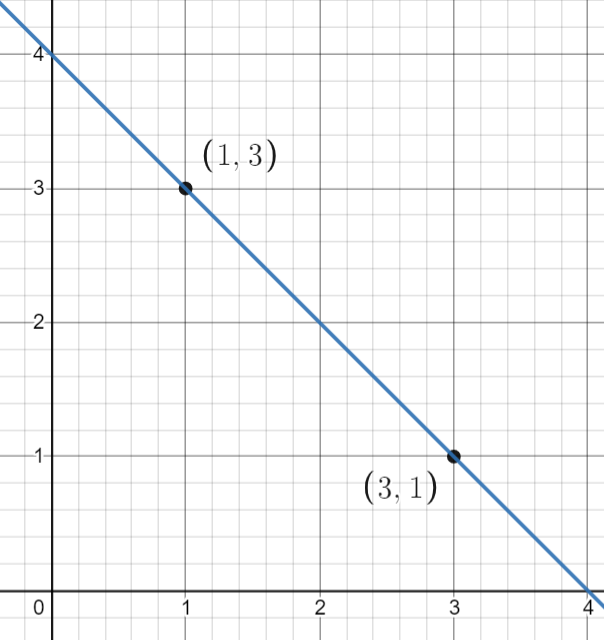# Slope Calculator

Enter Information
X1
Y1
X2
Y2

You can find the slope of a line and the quantities related to slope including the distance between two points, angle e.t.c using the slope formula calculator. This slope finder also provides a separate graph for every calculation.

## How to Use the Slope Calculator?

To use this slope calculator follow these steps.

1. Identify the values of $$X_1$$$$X_2$$, $$Y_1$$, and $$Y_2$$  from the available set of coordinates.
2. Enter these values in the labeled boxes.
3. Press enter or click calculate.

## What is Slope?

In literal terms, slope refers to the steepness and direction of a line. This term is used in coordinate geometry. The vector form of the slope is called gradient.The calculation of slope involves two pairs of coordinates. Each of these pairs has two values, one is of the horizontal axis while the other is of the vertical axis.

## Slope Formula:

For two general points: $$\left(X_1,Y_1\right)$$,$$\left(X_2,Y_2\right)$$, the slope calculator uses the following formula of the slope:

Slope = m = $$\dfrac{Rise}{Run}$$ = $$\dfrac{\Delta y}{\Delta x}$$

= Change in Y / Change in X

= $$\left(Y_2-\:Y_1\right),\left(X_2-X_1\right)$$

This formula tells us that slope is the ratio of the difference between vertical coordinates and the difference between horizontal coordinates.

## How to Find the Slope of a Line?

### Example:

Consider the following set of points;

$$\left(2,6\right)$$,$$\left(6,2\right)$$

Find the slope of the line passing through these points.

### Solution:

Step 1: Identify the values.

$$X_1$$ = 2

$$X_2$$ = 6

$$Y_1$$ = 6

$$Y_2$$ = 2

Step 2: By Use the slope formula:

Slope= Change in Y / Change in X

Slope = $$\left(Y_2-\:Y_1\right)/\left(X_2-X_1\right)$$

Slope = $$\left(2-\:6\right)/\left(6-2\right)$$

Slope = -1

You can also use our point slope form calculator to calculate the point-slope form between two points

## FAQ

### What is the Slope of a Line?

The gradient is also called as the slope of a line. The direction of any line or the value of steepness in the coordinate plane is defined as the line of a slope. There are different methods to calculate it. You can calculate it by inserting the given equations into the formula or by the coordinates of the points which are lying on a given straight line.

### How to Find the Slope of a Line with Two Points?

You can simply use the slope formula for this. It is m = y2-y1/x2-x1. You only need to enter the correct values of both coordinates of points to find the slope of a line with two points. Or you can also use our Slope Calculator to get step-by-step detailed solutions with graphs.

### What is the Slope of a Vertical Line?

If the value of x is undefined, negative, or infinitely large, then the slope is undefined and called vertical lines.

### What is the Slope of a Horizontal Line?

If the value of y is same with the given two points, then it is called the slope of a horizontal line. You can use slope formula to calculate it.

### Explain the formula of the y2-y1/x2-x1 calculator.

This formula is used to find the slope of a line with two points. X1 and y1 represent the first point of coordinates. While x2 and y2 represent the second point of coordinates. By using a slope formula calculator, you can calculate the slope of horizontal and vertical lines with graphs and points.

### What is Slope Formula?

m=y2-y1/x2-x1

Where

• m = Value of Slope.
• (x1,y1) = coordinates of the first point of the line
• (x2,y2) = coordinates of the second point of the line
Learn and Practice Slope | Math.net
Position-time graphs: Determining the slope of the line | physicsclassroom.com
Other Languages
User Ratings
• Total Reviews 0
• Overall Rating 0/5
• Stars
Reviews
No Review Yet